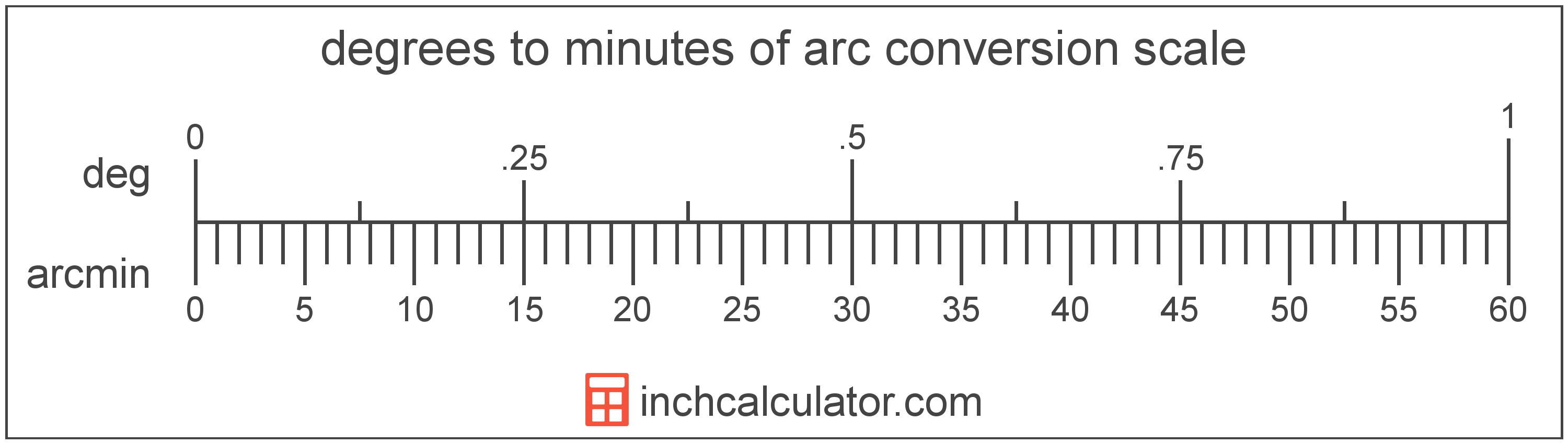# Minutes of Arc to Degrees Converter

Enter the angle in minutes of arc below to get the value converted to degrees.

Results in Degrees:1 arcmin = 0.016667°
1 arcmin = 0° 1′ 0″

Do you want to convert degrees to minutes of arc?

## How to Convert Minutes of Arc to Degrees

To convert a measurement in minutes of arc to a measurement in degrees, divide the angle by the following conversion ratio: 60 minutes of arc/degree.

Since one degree is equal to 60 minutes of arc, you can use this simple formula to convert:

degrees = minutes of arc ÷ 60

The angle in degrees is equal to the angle in minutes of arc divided by 60.

For example, here's how to convert 5 minutes of arc to degrees using the formula above.
degrees = (5' ÷ 60) = 0.083333°### Uses for Degrees, Minutes, & Seconds

For measurements in cartography and astronomy, degrees are often expressed in decimal form or degrees, minutes, and seconds (sometimes called DMS). Minutes and seconds are used to express portions of angle smaller than one degree.

You can use our DMS to degrees calculator to convert an angle to decimal form.

## What is a Minute of Arc?

The minute of arc is a unit of angle equal to 1/60th of one degree, or 1/21,600 of a circle. The minute of arc is also equal to π/10,800 radians.

A minute of arc is sometimes also referred to as an arc minute, arcminute, or minute arc. Minutes of arc can be abbreviated as arcmin, and are also sometimes abbreviated as MOA or amin. For example, 1 minute of arc can be written as 1 arcmin, 1 MOA, or 1 amin.

The minute of arc is most commonly represented using the prime (′), although the single-quote is commonly used. For instance, 1 minute of is most commonly expressed as 1′.

## What is a Degree?

A degree is a measure of angle equal to 1/360th of a revolution, or circle. The number 360 has 24 divisors, making it a fairly easy number to work with. There are also 360 days in the Persian calendar year, and many theorize that early astronomers used 1 degree per day.

The degree is an SI accepted unit for angle for use with the metric system. A degree is sometimes also referred to as a degree of arc, arc degree, or arcdegree. Degrees can be abbreviated as °, and are also sometimes abbreviated as deg. For example, 1 degree can be written as 1° or 1 deg.

Degrees can also be expressed using arcminutes and arcseconds as an alternative to using the decimal form. Arcminutes and arcseconds are expressed using the prime (′) and double-prime (″) characters, respectively, although a single-quote and double-quote are often used for convenience.

One arcminute is equal to 1/60th of a degree, and one arcsecond is equal to 1/60th of an arcminute.

Protractors are commonly used to measure angles in degrees. They are semi-circle or full-circle devices with degree markings allowing a user to measure an angle in degrees. Learn more about how to use a protractor or download a printable protractor.

## Minute of Arc to Degree Conversion Table

Table showing various minute of arc measurements converted to degrees.
Minutes Of Arc Degrees
1' 0.016667°
2' 0.033333°
3' 0.05°
4' 0.066667°
5' 0.083333°
6' 0.1°
7' 0.116667°
8' 0.133333°
9' 0.15°
10' 0.166667°
11' 0.183333°
12' 0.2°
13' 0.216667°
14' 0.233333°
15' 0.25°
16' 0.266667°
17' 0.283333°
18' 0.3°
19' 0.316667°
20' 0.333333°
21' 0.35°
22' 0.366667°
23' 0.383333°
24' 0.4°
25' 0.416667°
26' 0.433333°
27' 0.45°
28' 0.466667°
29' 0.483333°
30' 0.5°
31' 0.516667°
32' 0.533333°
33' 0.55°
34' 0.566667°
35' 0.583333°
36' 0.6°
37' 0.616667°
38' 0.633333°
39' 0.65°
40' 0.666667°

## References

1. Collins Dictionary, Definition of 'degree', https://www.collinsdictionary.com/us/dictionary/english/degree